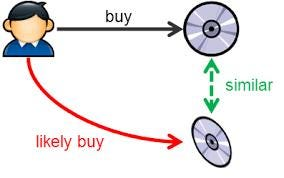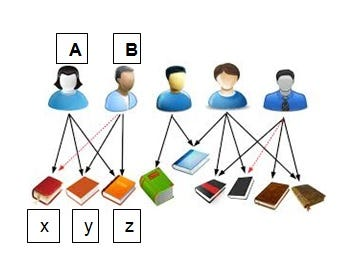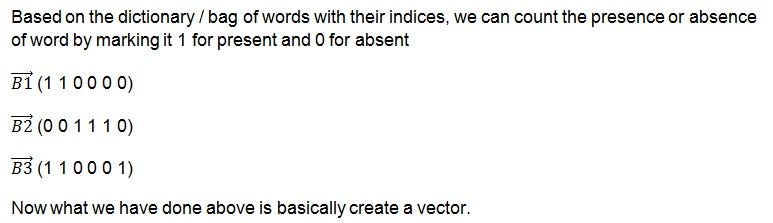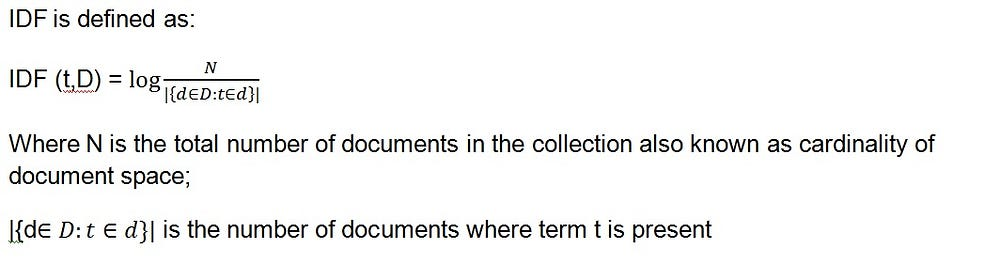#Subscribe to DSC Newsletter

Many of us are bombarded with various recommendations in our day to day life, be it on e-commerce sites or social media sites. Some of the recommendations look relevant but some create range of emotions in people, varying from confusion to anger.

There are basically two types of recommender systems, Content based and Collaborative filtering. Both have their pros and cons depending upon the context in which you want to use them.

Content based: In content based recommender systems, keywords or properties of the items are taken into consideration while recommending an item to an user. So, in a nutshell it is like recommending similar items. Imagine you are reading a book on data visualization and want to look for other books on the same topic. In this scenario, content based recommender system would be apt.Collaborative Filtering: Well to drive home the point, the below picture is the best example. Customer A has bought books x,y,z and customer B has bought books y,z. Now collaborative filtering technique would recommend book x to customer B. This is both the advantage and disadvantage of collaborative filtering. It does not matter if the book x was a nonfiction book while the liking of customer B was strictly fiction book. The relevancy of the recommendation may or may not be correct. Typically many companies use this technique since it allows them to cross sell products.Developing a Content Based Book Recommender System — Theory

Imagine you have a collection of data science books in your library and let’s say your friend has read a book on neural network and wants to read another book on the same topic to build up his/her knowledge on the subject. The best way is to implement a simple content based recommender system.

We will look at three important concepts here which go into building this content based recommender system.

• Vectors
• TF-IDF
• Cosine Similarity

Vectors

The fundamental idea is to convert the texts or words into a vector and represent in a vector space model. This idea is so beautiful and in an essence this very idea of vectors is what is making the rapid strides in Machine learning and AI possible. In fact Geoffrey Hinton (“Father of Deep Learning”) in a MIT technology review article acknowledged that the AI institute at Toronto has been named “Vector Institute” owing to the beautiful properties of vectors that has helped them in the field of Deep Learning and other variants of Neural nets.

TF — IDF

TF- IDF stands for Term Frequency and Inverse Document Frequency .TF-IDF helps in evaluating importance of a word in a document.

TF — Term FrequencyIn order to ascertain how frequent the term/word appears in the document and also to represent the document in vector form, let’s break it down to following steps.

Step 1: Create a dictionary of words (also known as bag of words) present in the whole document space. We ignore some common words also called as stop words e.g. the, of, a, an, is etc, since these words are pretty common and it will not help us in our goal of choosing important words

In this current example I have used the file ‘test1.csv’ which contains titles of 50 books. But to drive home the point, just consider 3 book titles (documents) to be making up the whole document space. So B1 is one document, B2 and B3 are other documents. Together B1, B2, B3 make up the document space.

B1 — Recommender Systems

B2 — The Elements of Statistical Learning

B3 — Recommender Systems — Advanced

Now creating an index of these words (stop words ignored)

1. Recommender 2. Systems 3 Elements 4. Statistical 5.Learning 6. Advanced

Step 2: Forming the vectorThe Term Frequency helps us identify how many times the term or word appears in a document but there is also an inherent problem, TF gives more importance to words/ terms occurring frequently while ignoring the importance of rare words/terms. This is not an ideal situation as rare words contain more importance or signal. This problem is resolved by IDF.

Sometimes a word / term might occur more frequently in longer documents than shorter ones; hence Term Frequency normalization is carried out.

TFn = (Number of times term t appears in a document) / (Total number of terms in the document), where n represents normalized.

IDF (Inverse Document Frequency):In some variation of the IDF definition, 1 is added to the denominator so as to avoid a case of division by zero in the case of no terms being present in the document.

Basically a simple definition would be:

IDF = ln (Total number of documents / Number of documents with term t in it)

Now let’s take an example from our own dictionary or bag of words and calculate the IDFs

We had 6 terms or words which are as follows

1. Recommender 2. Systems 3 Elements 4. Statistical 5.Learning 6. Advanced

and our documents were :

B1 — Recommender Systems

B2 — The Elements of Statistical Learning

B3 — Recommender Systems — Advanced

Now IDF (w1) = log 3/2; IDF(w2) = log 3/2; IDF (w3) = log 3/1; IDF (W4) = log 3/1; IDF (W5) = log 3/1; IDF(w6) = log 3/1

(note : natural logarithm being taken and w1..w6 denotes words/terms)

We then again get a vector as follows:

= (0.4054, 0.4054, 1.0986, 1.0986, 1.0986, 1.0986)

TF-IDF Weight:

Now the final step would be to get the TF-IDF weight. The TF vector and IDF vector are converted into a matrix.

Then TF-IDF weight is represented as:

TF-IDF Weight = TF (t,d) * IDF(t,D)

This is the same matrix which we get by executing the below python code:

`tfidf_matrix = tf.fit_transform(ds['Book Title'])`

Cosine Similarity:

Well cosine similarity is a measure of similarity between two non zero vectors. One of the beautiful thing about vector representation is we can now see how closely related two sentence are based on what angles their respective vectors make.

Cosine value ranges from -1 to 1.

So if two vectors make an angle 0, then cosine value would be 1, which in turn would mean that the sentences are closely related to each other.

If the two vectors are orthogonal, i.e. cos 90 then it would mean that the sentences are almost unrelated.

Developing a Content Based Book Recommender System — Implementation

Below I have written a few lines of code in python to implement a simple content based book recommender system. I have added comments (words after #) to make it clear what each line of code is doing.

```import pandas as pdfrom sklearn.feature_extraction.text import TfidfVectorizer
from sklearn.metrics.pairwise import cosine_similarity
ds = pd.read_csv("test1.csv") #you can plug in your own list of products or movies or books here as csv file
tf = TfidfVectorizer(analyzer='word', ngram_range=(1, 3), min_df=0, stop_words='english')
######ngram (1,3) can be explained as follows#####
#ngram(1,3) encompasses uni gram, bi gram and tri gram
#consider the sentence "The ball fell"
#ngram (1,3) would be the, ball, fell, the ball, ball fell, the ball fell```
`tfidf_matrix = tf.fit_transform(ds['Book Title'])cosine_similarities = cosine_similarity(tfidf_matrix,tfidf_matrix)`
`results = {} # dictionary created to store the result in a dictionary format (ID : (Score,item_id))`
```for idx, row in ds.iterrows(): #iterates through all the rows# the below code 'similar_indice' stores similar ids based on cosine similarity. sorts them in ascending order. [:-5:-1] is then used so that the indices with most similarity are got. 0 means no similarity and 1 means perfect similarity
similar_indices = cosine_similarities[idx].argsort()[:-5:-1] #stores 5 most similar books, you can change it as per your needs
similar_items = [(cosine_similarities[idx][i], ds['ID'][i]) for i in similar_indices]
results[row['ID']] = similar_items[1:]

#below code 'function item(id)' returns a row matching the id along with Book Title. Initially it is a dataframe, then we convert it to a list
def item(id):
return ds.loc[ds['ID'] == id]['Book Title'].tolist()
def recommend(id, num):
if (num == 0):
print("Unable to recommend any book as you have not chosen the number of book to be recommended")
elif (num==1):
print("Recommending " + str(num) + " book similar to " + item(id))

else :
print("Recommending " + str(num) + " books similar to " + item(id))

print("----------------------------------------------------------")
recs = results[id][:num]
for rec in recs:
print("You may also like to read: " + item(rec) + " (score:" + str(rec) + ")")```
```#the first argument in the below function to be passed is the id of the book, second argument is the number of books you want to be recommendedrecommend(5,2)

```

The Output

```Recommending 2 books similar to The Elements of Statistical Learning ----------------------------------------------------------
You may also like to read: An introduction to Statistical Learning  (score:0.389869522721)
You may also like to read: Statistical Distributions (score:0.13171009673)```

The list of id and book title in test1.csv are as below (20 rows shown)

```ID,Book Title1,Probabilistic Graphical Models
2,Bayesian Data Analysis
3,Doing data science
4,Pattern Recognition and Machine Learning
5,The Elements of Statistical Learning
6,An introduction to Statistical Learning
7,Python Machine Learning
8,Natural Langauage Processing with Python
9,Statistical Distributions
10,Monte Carlo Statistical Methods
11,Machine Learning :A Probablisitic Perspective
12,Neural Network Design
13,Matrix methods in Data Mining and Pattern recognition
14,Statistical Power Analysis
15,Probability Theory The Logic of Science
16,Introduction to Probability
17,Statistical methods for recommender systems
18,Entropy and Information theory
19,Clever Algorithms: Nature-Inspired Programming Recipes
20,"Precision: Principles, Practices and Solutions for the Internet of Things"

```

Now that you have read this article you may also like to read……..(well never mind ;) )

The same article is also available on the following links :

How To Build a Simple Content Based Book Recommender System

Medium: Recommender Engine - Under The Hood

Sources :

TF-IDF

Kaggle

Vector space model

Deeplearning4j

Sklearn Cosine Similarity

Sklearn TFidfvectorizer

Mark Needham Blog

MIT Technology Review

Views: 1771

Comment

### You need to be a member of Data Science Central to add comments!

Join Data Science Central

## Videos

• ### DSC Webinar Series: How to Use Time Series Data to Forecast at Scale

Added by Tim Matteson

• ### DSC Webinar Series: Why Data Prep is Step 1 for Analytics Success

Added by Tim Matteson

• ### DSC Webinar Series: 9 Best Practices for Data Blending

Added by Tim Matteson